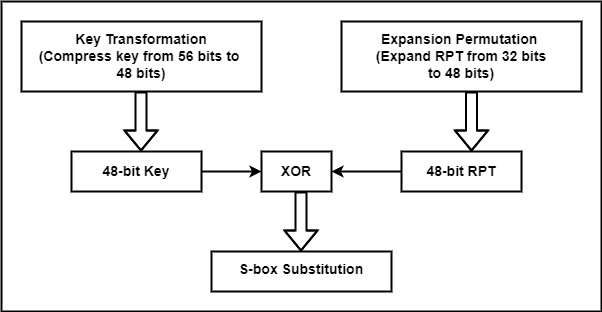# What is Expansion Permutation in Information Security?

This operation expands the right half of the information, R from 32 bits to 48 bits and this operation changes the order of the bits as well as repeating specific bits. It is called an expansion permutation.

This operation has two objective including it creates the right half the similar size as the key for the XOR operation and it supports a longer result that can be compressed during the substitution operation. It can be enabling one bit influence two substitutions, the dependency of the output bits on the input bits spread quicker. This is known as avalanche effect.

DES is designed to reach the action of having each bit of the ciphertext based on each bit of the plaintext and each bit of the key as rapidly as possible. This appears as follows −

• The 32-bit Right Plain Text is divided into eight blocks, with each blocks including 4 bits.

• Next each 4-bit block of the following process is expanded to a corresponding 6-bit block. That is per 4-bit block, 2 extra bits are inserted. They are absolutely the repeated first and fourth bits of the 4-bit block.

The second and the third bits are written down as they were in the input. The first bit download is outputted to the second output position, and further renew in output position 48. Likewise, the 32th input bit is found in the 47th output position as well as in the first output position.

Clearly this phase results into expansion as well as permutation of the input bits while making the output. The first input bit goes into the second and the 48th output positions.

The second input bit goes into the third output position, etc. Consequently, it will detect that the expansion permutation has generally used the table display in the figure.

RPT expansion permutation table

 32 1 2 3 4 5 4 5 6 7 8 9 8 9 10 11 12 13 12 13 14 15 16 17 16 17 18 19 20 21 20 21 22 23 24 25 24 25 26 27 28 29 28 29 30 31 32 1

It can be view firstly the key-transformation phase compresses the 56-bit key to 48 bits. Thus the expansion permutation process expands the 32-bit RPT (Right Plain Text) to 48 bits. Now the 48 bit key is XORed with the 48-bit RPT, and the resulting output is given to the next step, which is the S-box substitution as display in the figure.After the expansion permutation, DES need the XOR operation on the expanded right section and the round key.